This generic plot method for survfit.stanjm objects will plot the estimated subject-specific or marginal survival function using the data frame returned by a call to posterior_survfit. The call to posterior_survfit should ideally have included an "extrapolation" of the survival function, obtained by setting the extrapolate argument to TRUE.

The plot_stack_jm function takes arguments containing the plots of the estimated subject-specific longitudinal trajectory (or trajectories if a multivariate joint model was estimated) and the plot of the estimated subject-specific survival function and combines them into a single figure. This is most easily understood by running the Examples below.

# S3 method for survfit.stanjm
plot(
x,
ids = NULL,
limits = c("ci", "none"),
xlab = NULL,
ylab = NULL,
facet_scales = "free",
ci_geom_args = NULL,
...
)

plot_stack_jm(yplot, survplot)

## Arguments

x A data frame and object of class survfit.stanjm returned by a call to the function posterior_survfit. The object contains point estimates and uncertainty interval limits for estimated values of the survival function. An optional vector providing a subset of subject IDs for whom the predicted curves should be plotted. A quoted character string specifying the type of limits to include in the plot. Can be one of: "ci" for the Bayesian posterior uncertainty interval for the estimated survival probability (often known as a credible interval); or "none" for no interval limits. An optional axis label passed to labs. A character string passed to the scales argument of facet_wrap when plotting the longitudinal trajectory for more than one individual. Optional arguments passed to geom_ribbon and used to control features of the plotted interval limits. They should be supplied as a named list. Optional arguments passed to geom_line and used to control features of the plotted survival function. An object of class plot.predict.stanjm, returned by a call to the generic plot method for objects of class predict.stanjm. If there is more than one longitudinal outcome, then a list of such objects can be provided. An object of class plot.survfit.stanjm, returned by a call to the generic plot method for objects of class survfit.stanjm.

## Value

The plot method returns a ggplot object, also of class plot.survfit.stanjm. This object can be further customised using the ggplot2 package. It can also be passed to the function plot_stack_jm.

plot_stack_jm returns an object of class bayesplot_grid that includes plots of the estimated subject-specific longitudinal trajectories stacked on top of the associated subject-specific survival curve.

posterior_survfit, plot_stack_jm, posterior_traj, plot.predict.stanjm

plot.predict.stanjm, plot.survfit.stanjm, posterior_predict, posterior_survfit

## Examples

# \donttest{
if (!exists("example_jm")) example(example_jm)

# Obtain subject-specific conditional survival probabilities
# for all individuals in the estimation dataset.
ps1 <- posterior_survfit(example_jm, extrapolate = TRUE)

# We then plot the conditional survival probabilities for
# a subset of individuals
plot(ps1, ids = c(7,13,15))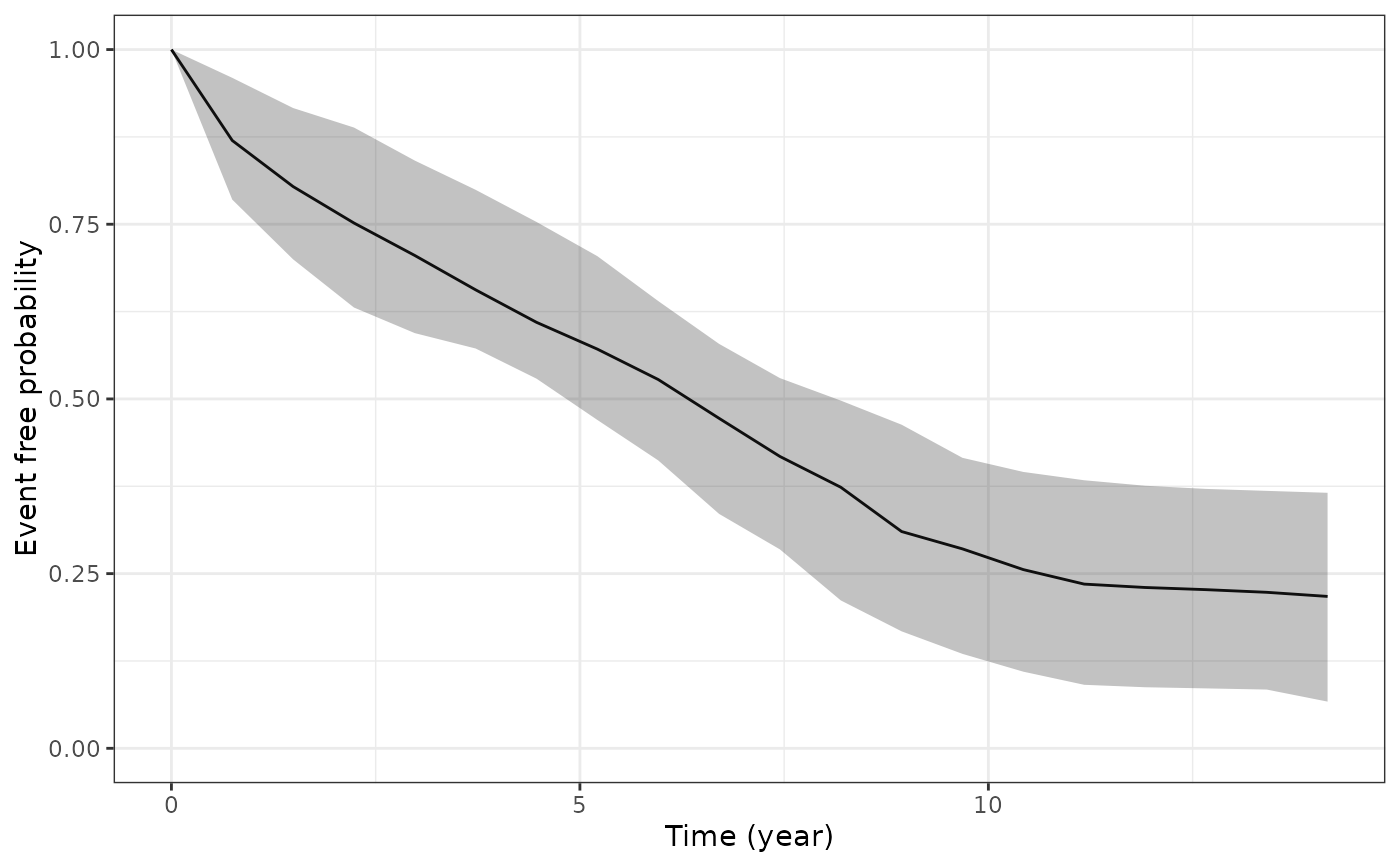# We can change or add attributes to the plot
plot(ps1, ids = c(7,13,15), limits = "none")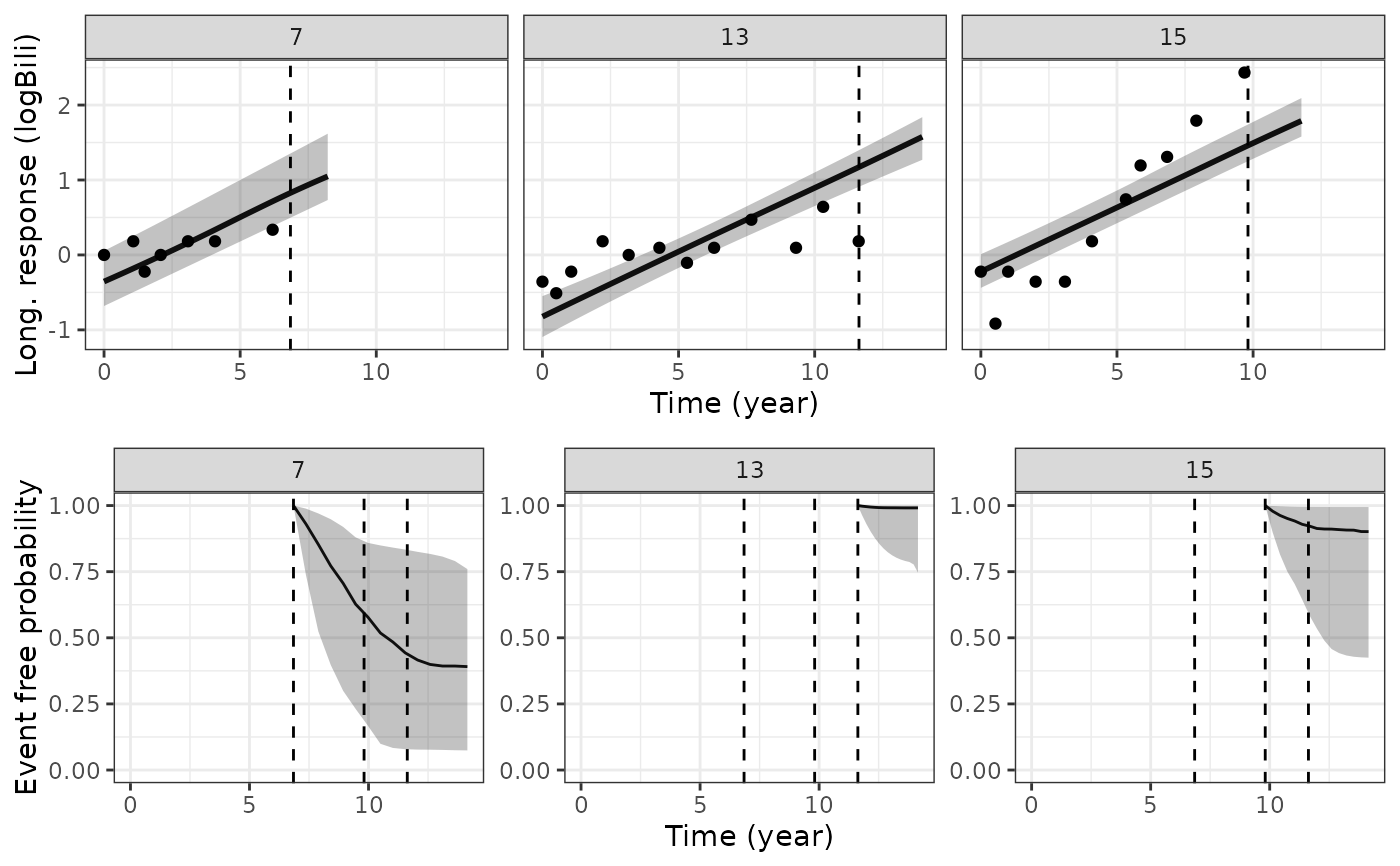plot(ps1, ids = c(7,13,15), xlab = "Follow up time")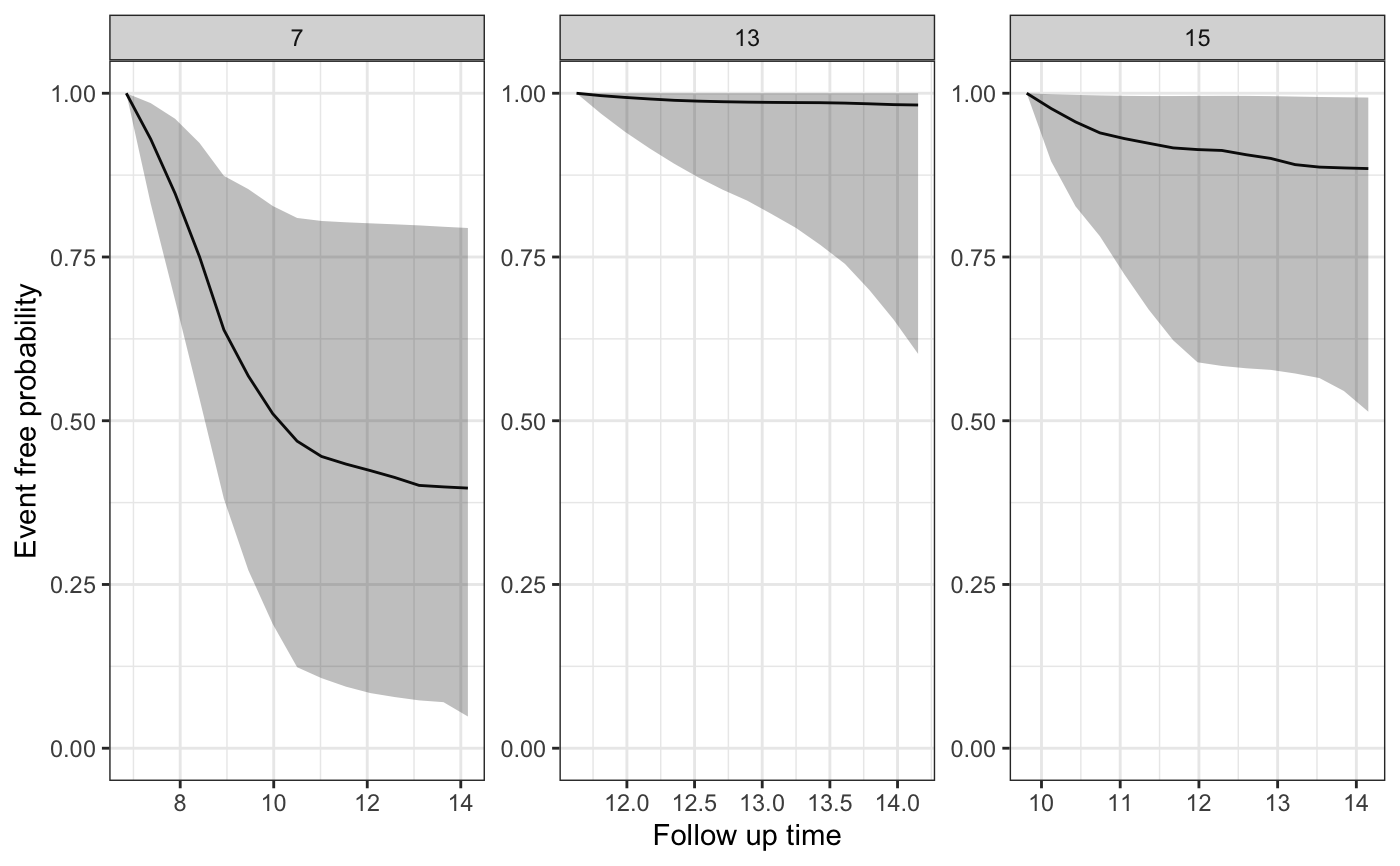plot(ps1, ids = c(7,13,15), ci_geom_args = list(fill = "red"),
color = "blue", linetype = 2)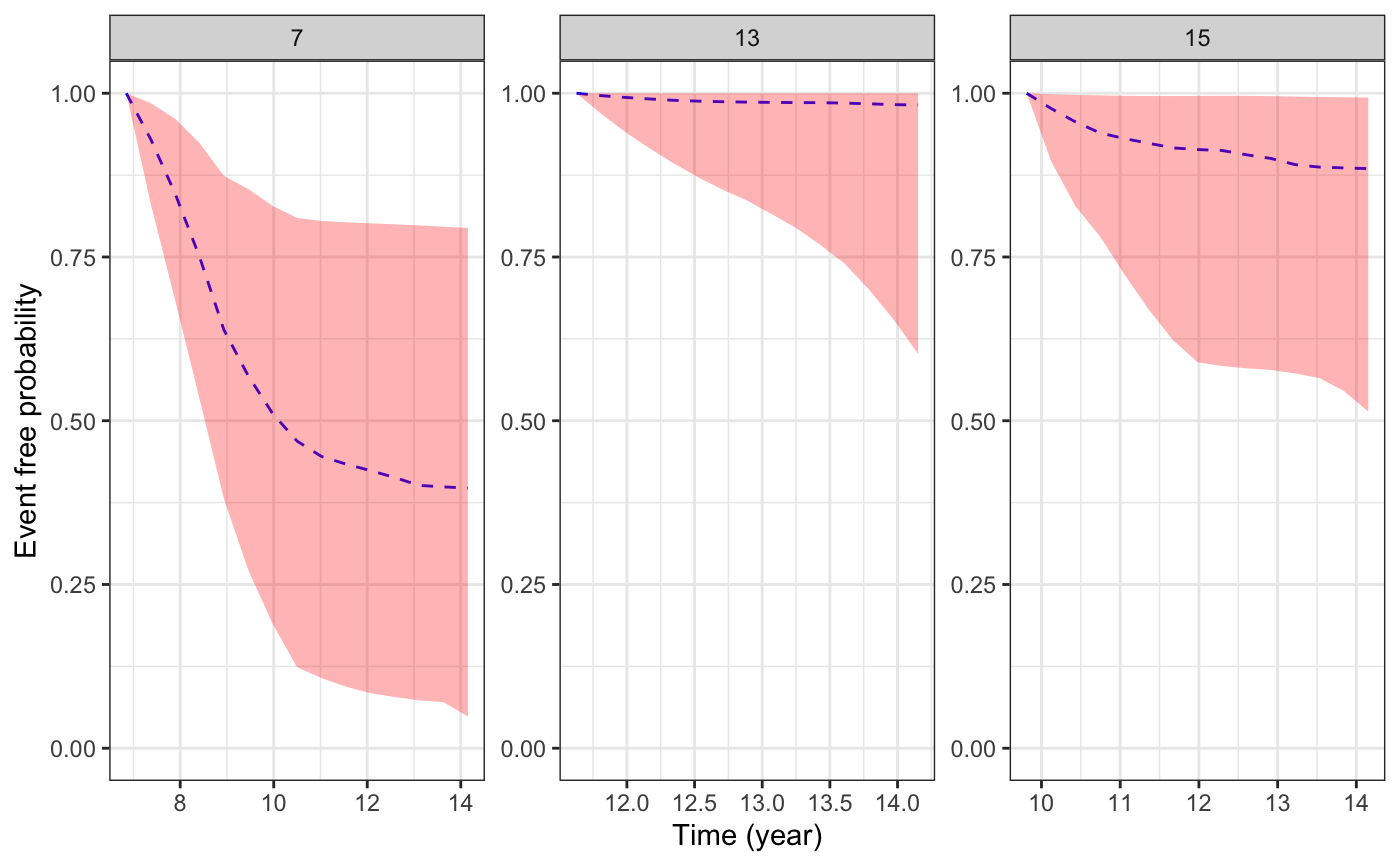plot(ps1, ids = c(7,13,15), facet_scales = "fixed")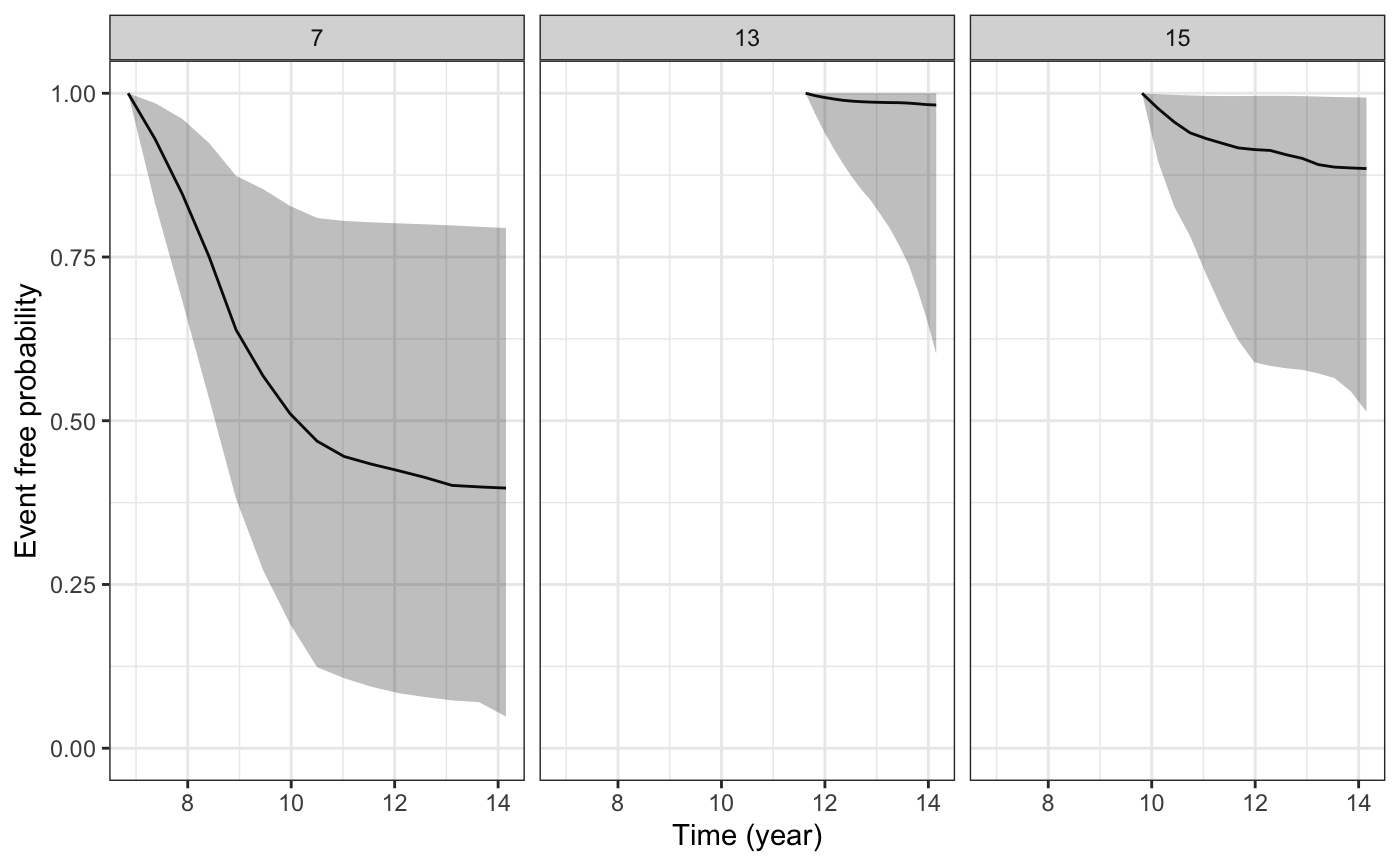# Since the returned plot is also a ggplot object, we can
# modify some of its attributes after it has been returned
plot1 <- plot(ps1, ids = c(7,13,15))
plot1 +
ggplot2::theme(strip.background = ggplot2::element_blank()) +
ggplot2::coord_cartesian(xlim = c(0, 15)) +
ggplot2::labs(title = "Some plotted survival functions")#> Coordinate system already present. Adding new coordinate system, which will replace the existing one.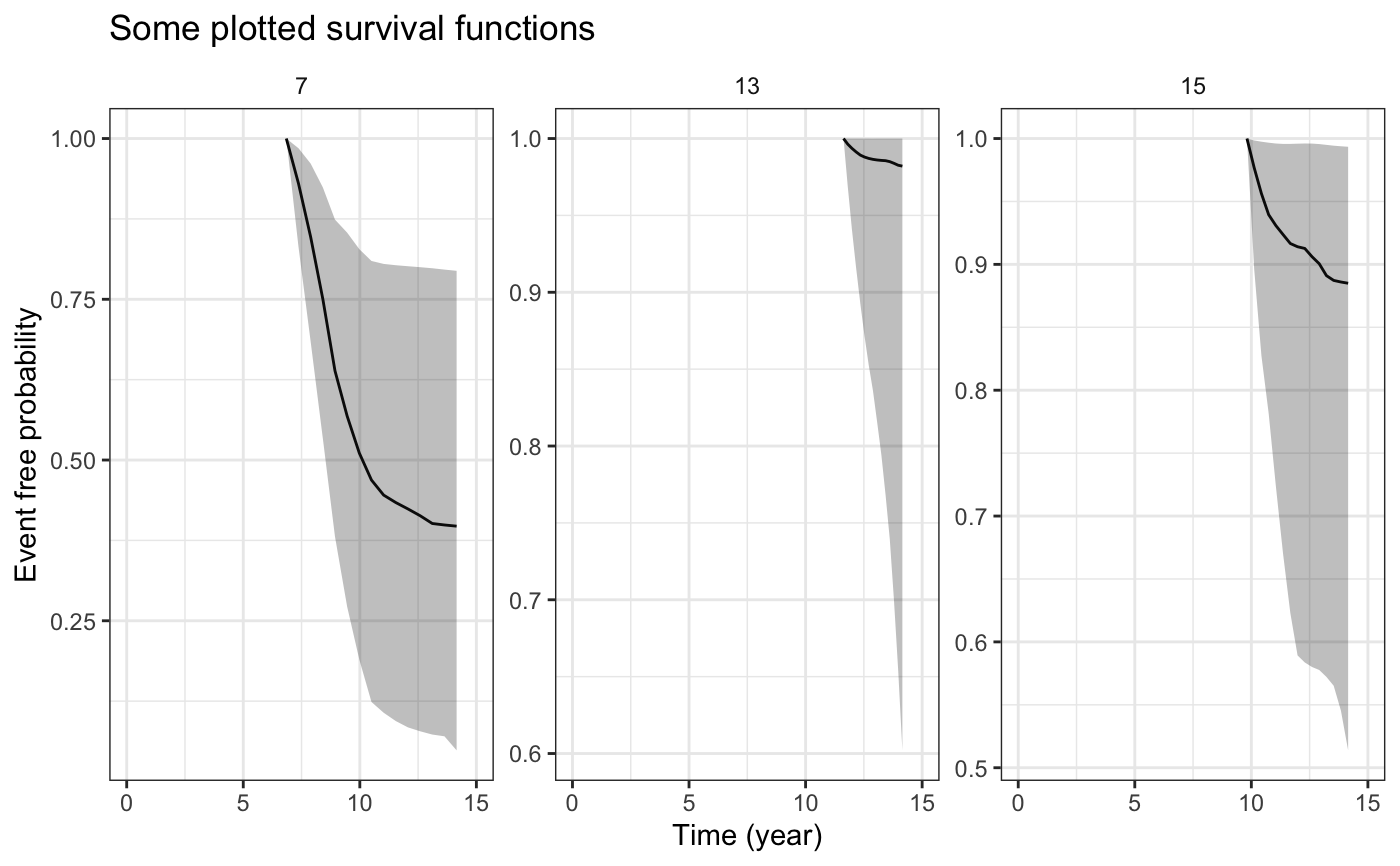# We can also combine the plot(s) of the estimated
# subject-specific survival functions, with plot(s)
# of the estimated longitudinal trajectories for the
# same individuals
ps1 <- posterior_survfit(example_jm, ids = c(7,13,15))
pt1 <- posterior_traj(example_jm, , ids = c(7,13,15))
plot_surv <- plot(ps1)
plot_traj <- plot(pt1, vline = TRUE, plot_observed = TRUE)#> geom_smooth() using formula 'y ~ x'#> geom_smooth() using formula 'y ~ x'  plot_stack_jm(plot_traj, plot_surv)#> geom_smooth() using formula 'y ~ x'#> geom_smooth() using formula 'y ~ x'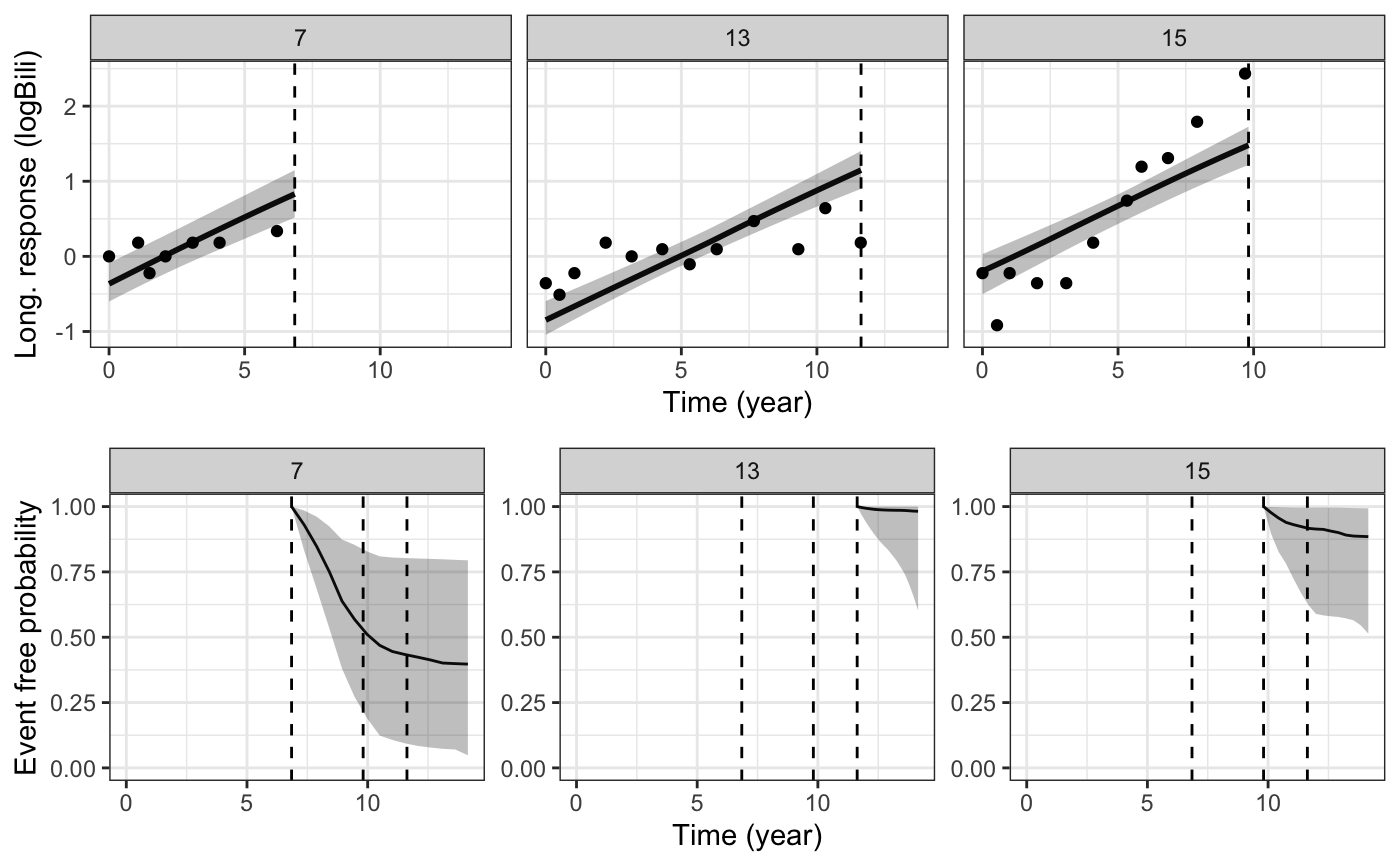# Lastly, let us plot the standardised survival function
# based on all individuals in our estimation dataset
ps2 <- posterior_survfit(example_jm, standardise = TRUE, times = 0,
control = list(epoints = 20))
plot(ps2)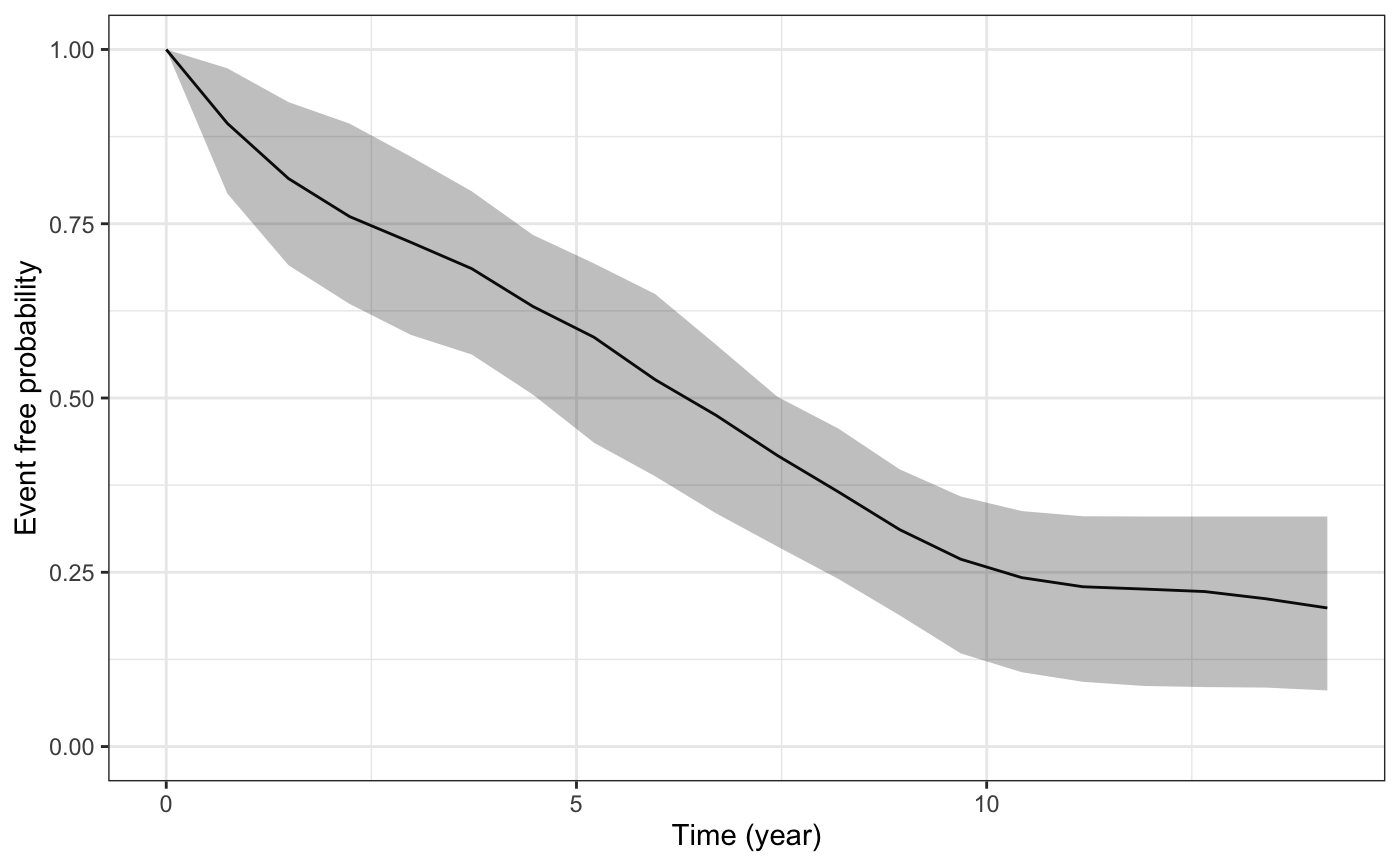# }

# \donttest{
if (!exists("example_jm")) example(example_jm)
ps1 <- posterior_survfit(example_jm, ids = c(7,13,15))
pt1 <- posterior_traj(example_jm, ids = c(7,13,15), extrapolate = TRUE)
plot_surv <- plot(ps1)
plot_traj <- plot(pt1, vline = TRUE, plot_observed = TRUE)#> geom_smooth() using formula 'y ~ x'#> geom_smooth() using formula 'y ~ x'  plot_stack_jm(plot_traj, plot_surv)#> geom_smooth() using formula 'y ~ x'#> geom_smooth() using formula 'y ~ x'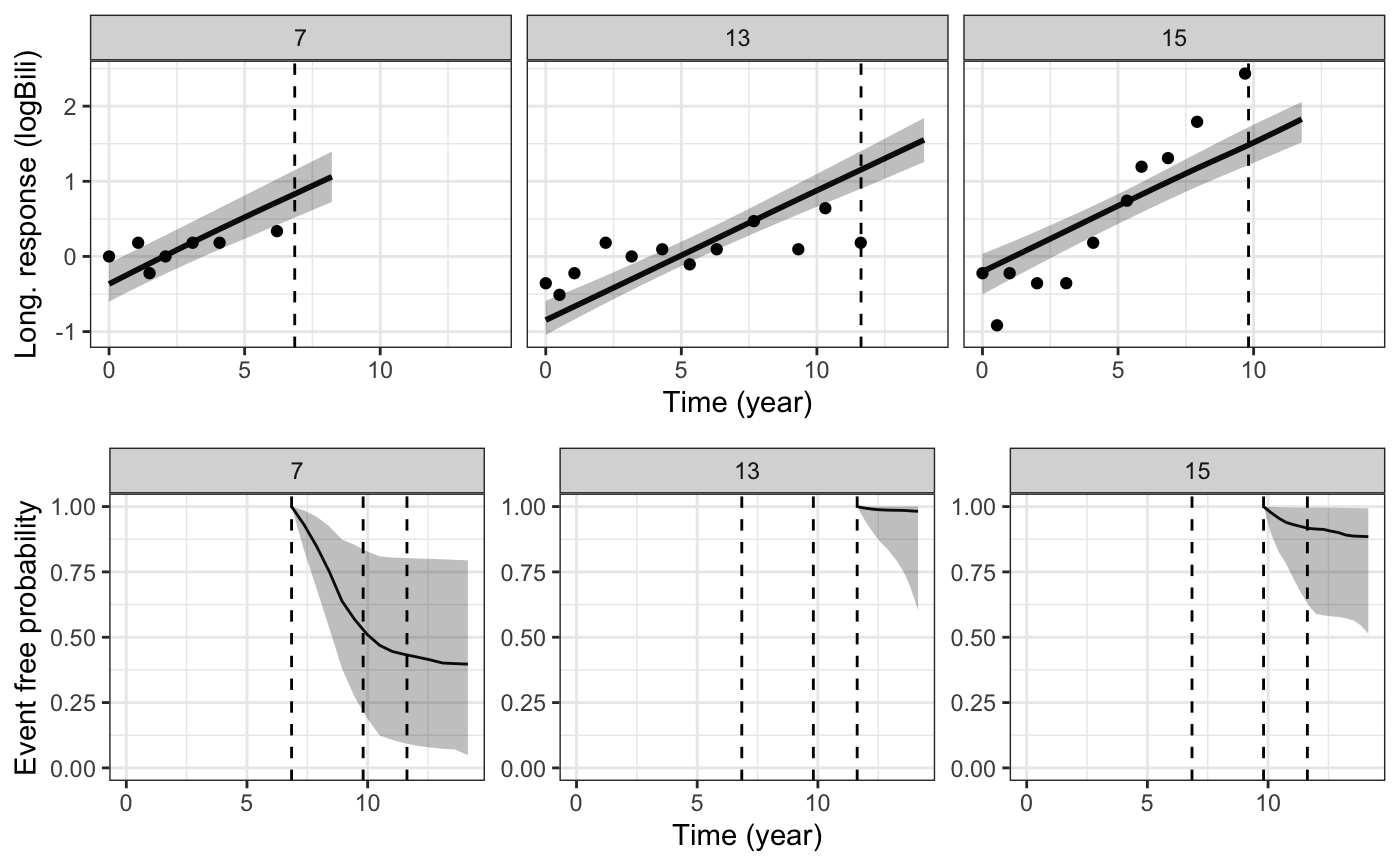# }